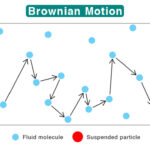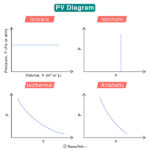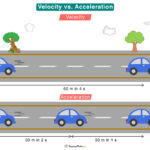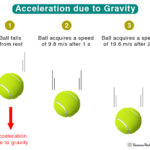Home / Physics / Types of Energy

# Types of Energy

Energy comes in many different forms. Since energy cannot be created or destroyed, it transforms from one form to another. The two main types of energy are kinetic energy and potential energy.

## Kinetic Energy

Kinetic energy is the energy associated with an object in motion. The motion can be translational, rotational, and vibrational. Kinetic energy can be of several types.

### 1. Motion Energy

Motion energy is the energy due to the movement of an object. The faster the object moves, the higher its energy is. Also, a heavier object has more energy than a lighter one.

Examples

• A person cycling
• A bullet moving through the air
• Wind howling

### 2. Electrical Energy

Electrical energy is due to the flow of electrons in a wire that results in current. It is used at home to power electrical appliances.

Examples

• Powering a television
• Turning on the lights
• Lightning

### 3. Sound Energy

Sound energy is due to the vibration of air particles. When these vibrations reach the ears, the brain perceives them as sound.

Examples

• Drumming of drums
• A balloon popping
• A plane flying

Radiant energy is due to oscillating electric and magnetic fields. It propagates in a direction perpendicular to the planes of oscillations. Particles or waves carry the energy.

Examples

• Light and heat from the Sun
• Radiation emitted by an X-ray machine
• Microwave emitted by a microwave oven

### 5. Thermal Energy

Thermal energy is because of the random motion of atoms and molecules in a substance. It is transferred as heat, which alters the temperature of the substance. The thermal energy present underneath the Earth’s surface is called Geothermal energy.

Examples

• Baking a pie in an oven
• A hot cup of coffee
• The heat from an electric heater

## Potential Energy

Potential energy is the energy due to an object’s relative position to a reference level. As the position changes, the object gains or loses potential energy. It is of several types.

### 1. Gravitational Potential Energy

Gravitational potential energy is due to the Earth’s gravitational force. It is the energy stored in an object due to its height from Earth’s surface. The greater the height, the higher the gravitational potential energy.

Examples

• An object suspended in the air
• A car on top of a hill
• An apple on a tree

### 2. Electric Potential Energy

Electric potential energy is due to the interaction between charged particles. A point charge in an electric field has potential energy.

Examples

• Capacitor

### 3. Elastic Potential Energy

Elastic potential energy is stored in an elastic material when compressed or stretched. When the energy is stored in the spring, it is called spring energy.

Examples

• A stretched rubber band
• An archer stretching a bow
• A person jumping on a trampoline

### 4. Chemical Energy

Chemical energy is the energy stored in the bonds of a chemical compound. It is released during a chemical reaction when the bond breaks.

Examples

• A battery powering a flashlight
• Gasoline fueling a vehicle
• Digesting food

### 5. Nuclear Energy

Nuclear energy is the energy inside the atoms that make up matter. It is released when atoms combine (nuclear fusion) or split (nuclear fission).

Examples

• The fusion reaction in the Sun
• Fission reaction in nuclear power plants to produce electricity
• Fission reaction in a nuclear bomb

Article was last reviewed on Wednesday, June 22, 2022

### Related articlesBrownian MotionPV DiagramVelocity vs AccelerationAcceleration due to Gravity

### One response to “Types of Energy”

1. Bulcha Gute says:

It is very interesting information about energy.